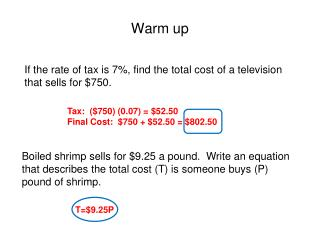Download PresentationWarm up

# Warm up

Download Presentation## Warm up

- - - - - - - - - - - - - - - - - - - - - - - - - - - E N D - - - - - - - - - - - - - - - - - - - - - - - - - - -
##### Presentation Transcript

1. If the rate of tax is 7%, find the total cost of a television that sells for \$750. Warm up Tax: (\$750) (0.07) = \$52.50 Final Cost: \$750 + \$52.50 = \$802.50 Boiled shrimp sells for \$9.25 a pound. Write an equation that describes the total cost (T) is someone buys (P) pound of shrimp. T=\$9.25P

2. Similarity – sides are proportional and angles are congruent. 10 8 5 4 6 3 Scale factor is 2 : 1

3. A tree is 32 feet tall. The tree casts a shadow of length 24 feet. If the shadow of a motel is 60 feet in length, how tall is the motel?

4. Tree: 32 24 Motel: x 60 A tree is 32 feet tall. The tree casts a shadow of length 24 feet. If the shadow of a motel is 60 feet in length, how tall is the motel?

5. A 42-foot tree casts a 27-foot shadow. At the same time, a flagpole casts a shadow 9 feet long. What is the flagpole’s height?

6. Tree: 42 27 Flagpole: x 9 A 42-foot tree casts a 27-foot shadow. At the same time, a flagpole casts a shadow 9 feet long. What is the flagpole’s height?

7. Which of the following is a true statement?A. ∆PQR =∆STUB. ∆PQR ≈ ∆STUC. ∆PQR is both similar & congruent to ∆STUD. ∆PQR is neither similar nor congruent to ∆STU. R U 3 4 8 6 S T 3 P 9 Q Answer: D

8. A 32 B 40 C x E 54 D

9. A 6 B 12 C 7 E x D

10. The sheet of paper on the left is a reduced copy of the original. What is the length of the copy to the nearest tenth of an inch? 8.5 in. 6 in. 11 in. x in.